# Civil Engineering - UPSC Civil Service Exam Questions

11.

Consider the following statements about concrete sleepers :
1. They improve the track modulus.
2. They have good scrap value.
3. They render transportation easy
4. They maintain the gauge quite satisfactorily.
Of these statements :

 A. 1 and 2 are correct B. 2 and 3 are correct C. 3 and 4 are correct D. 1 and 4 are correct

Explanation:

No answer description available for this question. Let us discuss.

12.

When are dropman holes provided in a sewerage system ?

 A. There is a change from gravity system to pressure system B. There is a change in the elevation of the ground level C. There is change in the diameter of the sewer D. There is change in the direction of the sewer line

Explanation:

No answer description available for this question. Let us discuss.

13.

Consider the following statements :
For the manufacture of good quality bricks it is essential to
1. use a reverberatory kiln.
2. blend the soil with clay or sand as deemed appropriate.
3. knead the soil in a ghani.
4. temper the soil in a pug mill.
Of these statements;

 A. 1 and 3 are correct B. 2 and 4 are correct C. 1, 3 and 4 are correct D. 2, 3 and 4 are correct

Explanation:

No answer description available for this question. Let us discuss.

14.

For the propped cantilever beam shown in the figure given above, the plastic moment capacity is Mp. What is the value of its collapse load ?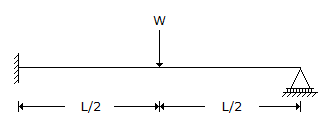A.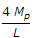B.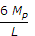C.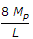D.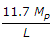Explanation:

No answer description available for this question. Let us discuss.

15.

Consider the following statements in case of impulse turbine :
1. The pressure and velocity both change as the water flows through the turbine.
2. Pressure throughout remains atmospheric and the velocity alone changes.
3. All the available energy is converted into kinetic energy.
4. Water is admitted over the entire circumference of the runner.
5. Water is admitted only through the nozzle.
Which of these statements are correct ?

 A. 2, 3 and 5 B. 2 and 3 C. 1, 4 and 5 D. 1 and 4